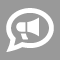#Blogs

## Word Problem Blogs

Newest Most Active

Often times experienced mathematicians tend to get comfortable with certain problem-solving strategies. For example, in a problem one might use a system of equations to solve a problem rather than employing a simpler more easy way to solve it. Though using system of equations are great, knowing how to solve problems using different approaches is important, not just for oneself, but for their students.   Take for example the following problem: A farmer has both pigs and chickens on his farm. There are 78 feet and 27 heads. How many pigs and how many chickens are there?   Solution 1: (Using Algebra System of Equations) 4p+2c=78 (pigs have 4 feet and chickens have 2 feet with 78 feet in total) p+c=27 (27 heads mean that the number of chicken and pigs total 27) Then by algebra p=27-c. Therefore by substitution, 4(27-c)+2c=78. 108-2c=78. 2c=30. c=15. Since, c=15, p+c=p+15=27. p=12. Therefore, the farmer has 15 chickens and 12 pigs... read more

Reading Formulas can make or break how a student comprehends their formula when alone - outside the presence of the teacher, instructor, tutor, or parent. Formula for Area of Circle:  A = π * r^2 Ineffective ways to read the area of a circle formula are as follows: Area is π times the radius squared. Area is π times the radius of the circle squared. Area of a circle is π times the radius squared. A equals π times r squared. >>>> Why are these ways NOT effective ways to read this formula? <<<<< 1.       Students will recall and repeat what they hear their educators say. 2.       If students recall letters (A) versus words (Area of a Circle) they will not realize the connection with word problems. 3.       Half way reading the formula (radius versus radius of a circle) creates empty pockets or disconnects in... read more

## Stuck?

Find the perfect tutor and raise your grades.